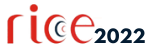# Proceedings of the 2022 Seventh International Conference on Research in Intelligent and Computing in Engineering

## An investigation of the complexities of a malignant tumor's fractional-order mathematical model

### Rakhi Singh, Vijay Kumar Gupta, Jyoti Mishra

Citation: Proceedings of the 2022 Seventh International Conference on Research in Intelligent and Computing in Engineering, Vu Dinh Khoa, Shivani Agarwal, Gloria Jeanette Rincon Aponte, Nguyen Thi Hong Nga, Vijender Kumar Solanki, Ewa Ziemba (eds). ACSIS, Vol. 33, pages 207211 ()

Full text

Abstract. In this paper, model of a malignant tumor \& associated problems are examined using fractional-order method. We consider a case where the malignant tumor cells' net death rate is solely time-dependent. Fractional homotopy decomposition method (HDM) has been applied to determine model's series solution. The answer to the HDM is given using the Maclaurin expansion. This method's use of the Mathematica software package allows for fast and simple computation of series solutions, which is one of its benefits.

### References

1. Atangana A, Alabaraoye E. Solving a system of fractional partial differential equations arising in the model of HIV infection of CD4+ cells and attractor one-dimensional Keller-Segel equations. Advances in Difference Equations. 2013 Dec;2013(1):1-4.
2. Gandhi H, Tomar A, Singh D. A predicted mathematical cancer tumor growth model of brain and its analytical solution by reduced differential transform method. In Proceedings of International Conference on Trends in Computational and Cognitive Engineering 2021 (pp. 203-213). Springer, Singapore.
3. Saadeh R, Qazza A, Amawi K. A New Approach Using Integral Transform to Solve Cancer Models. Fractal and Fractional. 2022 Aug 31;6(9):490.
4. Korpinar Z, Inc M, Hınçal E, Baleanu D. Residual power series algorithm for fractional cancer tumor models. Alexandria Engineering Journal. 2020 Jun 1;59(3):1405-12.
5. Iyiola OS, Zaman FD. A fractional diffusion equation model for cancer tumor. AIP Advances. 2014 Oct 13;4(10):107121.
6. Atangana A, Secer A. The time-fractional coupled-Korteweg-de-Vries equations. InAbstract and Applied Analysis 2013 Jan 1 (Vol. 2013). Hindawi.
7. Burgess PK, Kulesa PM, Murray JD, Alvord Jr EC. The interaction of growth rates and diffusion coefficients in a three-dimensional mathematical model of gliomas. Journal of Neuropathology & Experimental Neurology. 1997 Jun 1;56(6):704-13.
8. Moyo S, Leach PG. Symmetry methods applied to a mathematical model of a tumour of the brain. InProceedings of Institute of Mathematics of NAS of Ukraine 2004 (Vol. 50, No. Part 1, pp. 204-210).
9. Ali SM, Bokhari AH, Yousuf M, Zaman FD. A spherically symmetric model for the tumor growth. Journal of Applied Mathematics. 2014 Jan 1;2014.
10. Bokhari AH, Kara AH, Zaman FD. On the solutions and conservation laws of the model for tumor growth in the brain. Journal of Mathematical analysis and Applications. 2009 Feb 1;350(1):256-61.
11. El-Ajou A, Arqub OA, Zhour ZA, Momani S. New results on fractional power series: theories and applications. Entropy. 2013 Dec 2;15(12):5305-23.
12. El-Ajou A, Arqub OA, Momani S. Approximate analytical solution of the nonlinear fractional KdV–Burgers equation: a new iterative algorithm. Journal of Computational Physics. 2015 Jul 15;293:81-95.
13. Khater MM, Attia RA, Lu D. Numerical solutions of nonlinear fractional Wu–Zhang system for water surface versus three approximate schemes. Journal of Ocean Engineering and Science. 2019 Jun 1;4(2):144-8.
14. Atangana A, Botha JF. Analytical solution of the groundwater flow equation obtained via homotopy decomposition method. Journal of Earth Science & Climatic Change. 2012;3(2):115.
15. Atangana A, Baleanu D. Nonlinear fractional Jaulent-Miodek and Whitham-Broer-Kaup equations within Sumudu transform. InAbstract and applied analysis 2013 Jan 1 (Vol. 2013). Hindawi.